# Calculate

Calculate the area of a circle whose radius is 5 dm.

S =  78.5398 dm2

### Step-by-step explanation: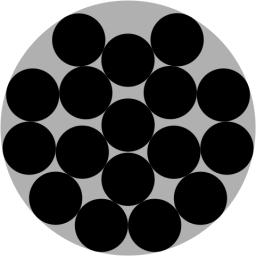Did you find an error or inaccuracy? Feel free to write us. Thank you!#### You need to know the following knowledge to solve this word math problem:

We encourage you to watch this tutorial video on this math problem:

## Related math problems and questions:

• Parallelogram - sideCalculate the length of the side of a parallelogram whose area is 175 dm2 and height to that side is 24.2 dm long.
• Surface and volumeCalculate the surface and volume of a cylinder whose height is 8 dm and the radius of the base circle is 2 dm
• Circle area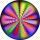Calculate the circle area with a radius of 1.2 m.
• Circle - easy 2The circle has a radius 6 cm. Calculate:
• AnnulusThe radius of the larger circle is 8cm, the radius of smaller is 5cm. Calculate the contents of the annulus.
• Rhombus HP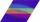Calculate the area of the rhombus with height 24 dm and perimeter 12 dm.
• Diameter to areaFind the area of a circle whose diameter is 26cm.
• Area of squareCalculate the content area of the square whose perimeter is 24 dm.
• Perimeter of the circleCalculate the perimeter of the circle in dm, whose radius equals the side of the square containing 0.49 dm2?
• AquariumCan 30 liters of water fit in a cuboid aquarium with dimensions a = 3dm b = 6dm c = 5dm?
• Diameter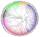The diameter of a circular plot is 14 dm. Find the circumference and area.Calculate the radius of the circle whose length is 107 cm larger than its diameterCalculate the radius of the quadrant, which area is equal to area of circle with radius r = 15 cm.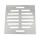The locksmith cut a square metal plate with a sidelong 6 dm into two identical rectangular openings with 2.5 dm and 2 dm dimensions. Calculate the remainder of the square plate.Find the radius of the circle with area S = 200 cm².Calculate approximately the area of a circle with radius 20 cm. When calculating π, use 22/7.Circle segment has a circumference of 135.26 dm and 2096.58 dm2 area. Calculate the radius of the circle and size of the central angle.